# Small PA driver for HF

Small PA driver for the Hermes-Lite Class-A HF PA.

Main characteristics:

• 15.5 dB gain
• 180 mW (22.7 dBm) output at 1 dB of compression
• 50 mW per output tone for an IM3 of -30 dBc with a two-tone input
• DC input power: Vdc=12.0 V, device bias current IccQ about 40 mA

The circuit is a slightly higher gain version of the similar driver #5, still a classical resistive feedback topology.
The base series resistor was removed, since it was found to be not needed for the amplifier stability.

The same circuit was actually built using two (quite) different transistors:

This is the driver schematic: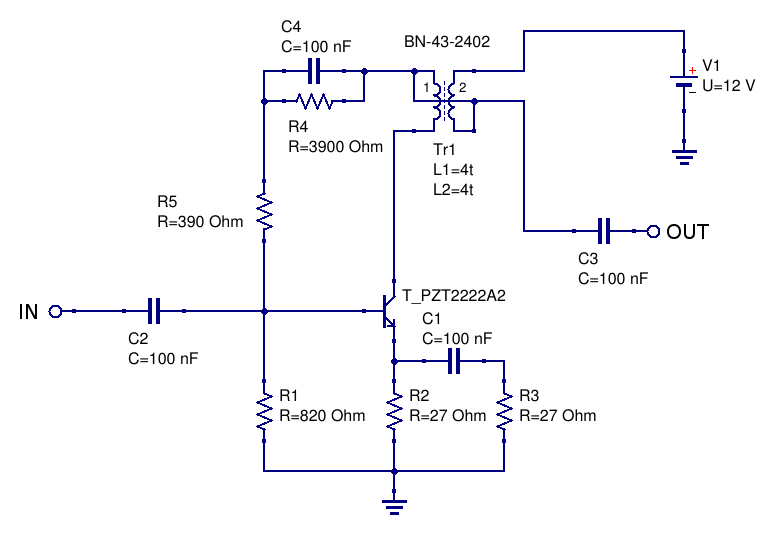## First version, with a 2N2222A

All measurement made with Vdc=12.0 V. IccQ=40 mA.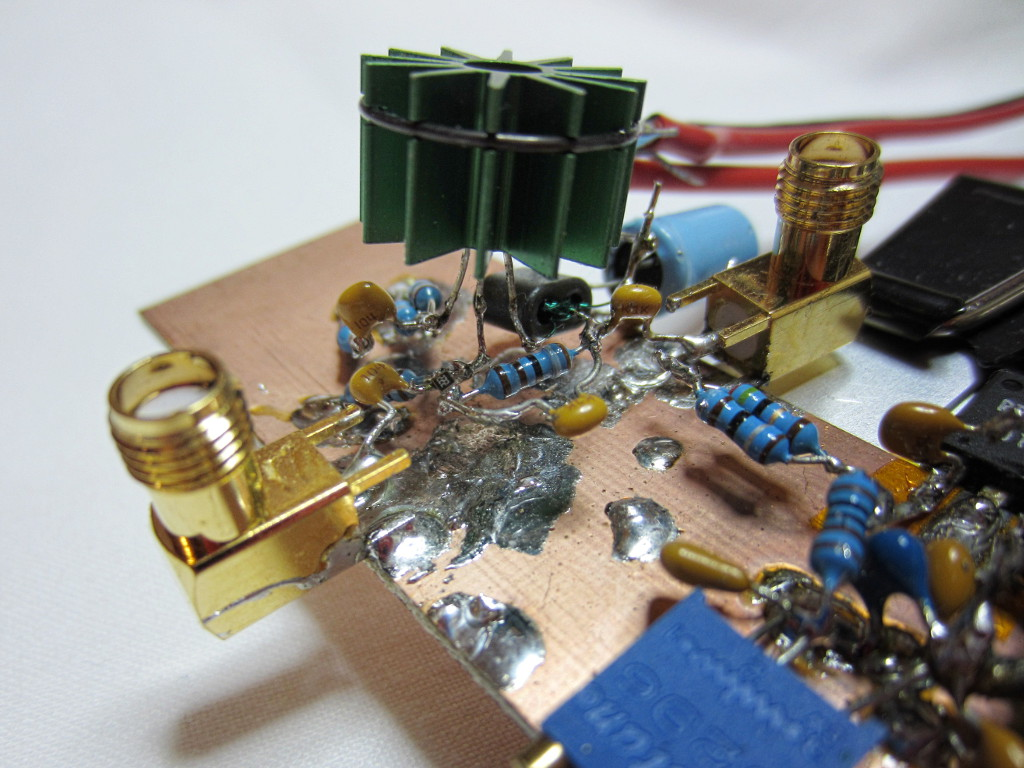Intermodulation products at 180 mW PEP output: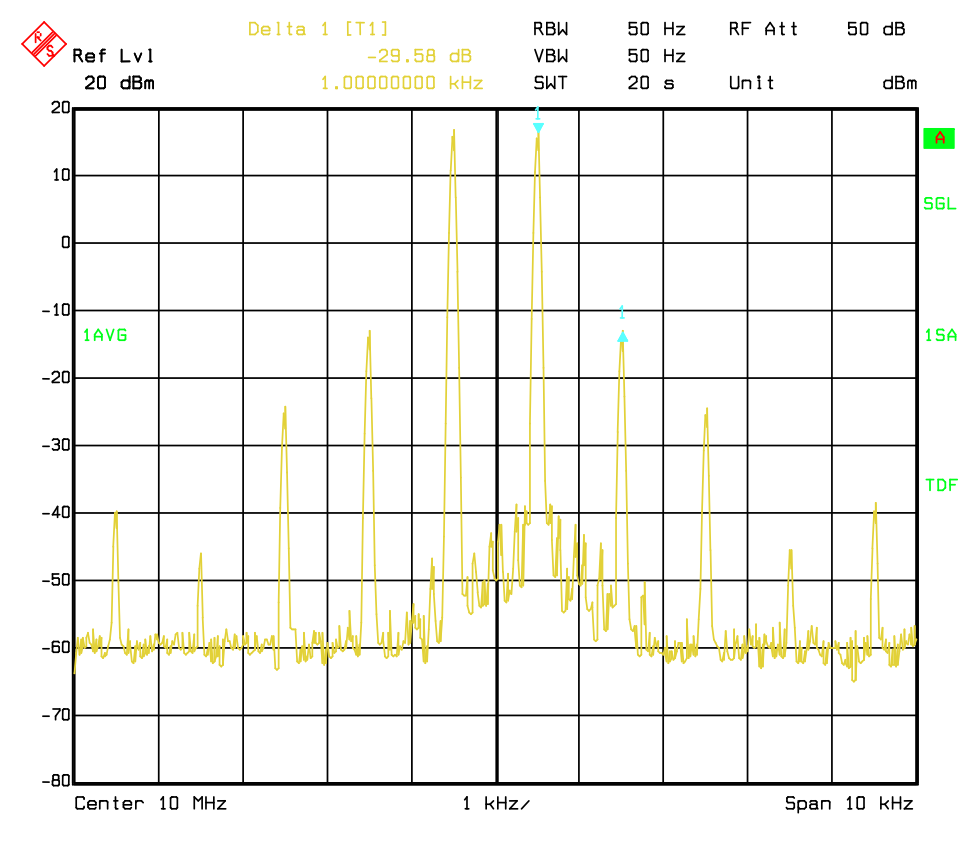Measured small-signal S-parameters (with -16 dBm input):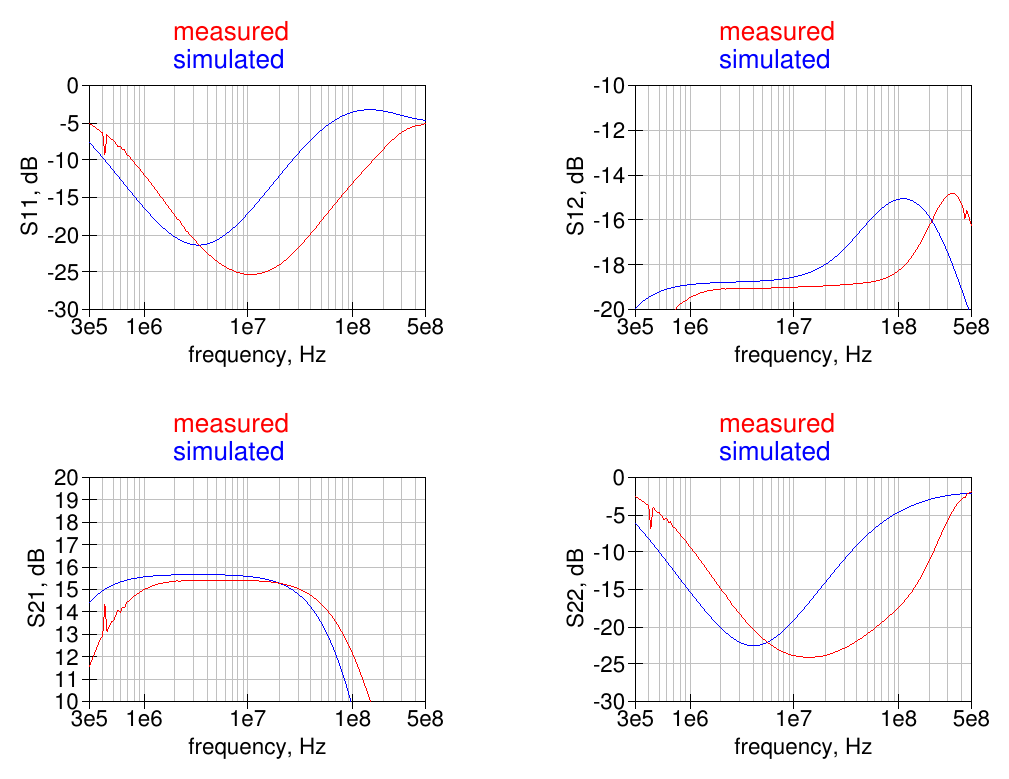.

The fundamental and harmonics output levels versus input power, with a 10 MHz sinewave input, is shown in the graph below: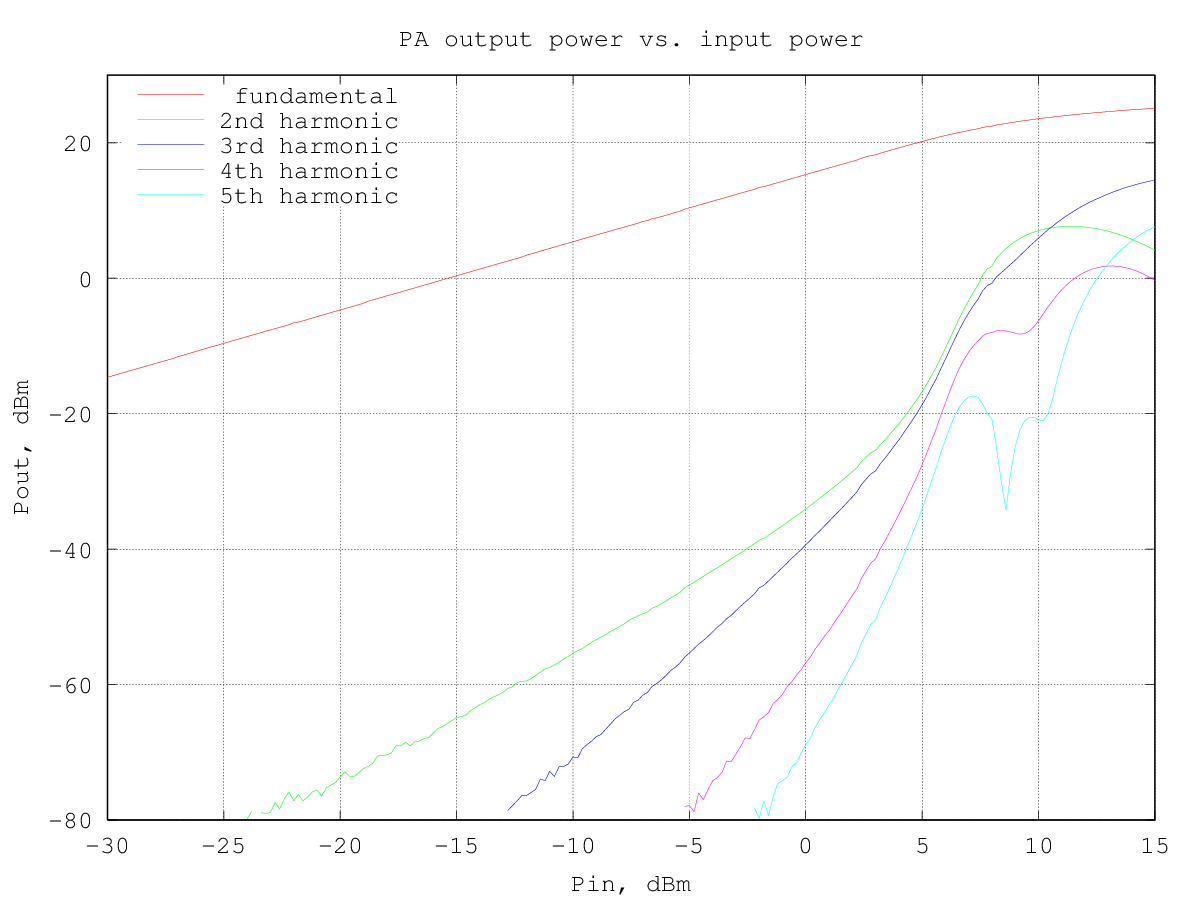the output power at 1 dB of compression is about 180 mW (22.7 dBm), as for the similar driver #5.

RF output and DC current drawn from the supply versus input power (10 MHz sinewave) are shown in the following graph: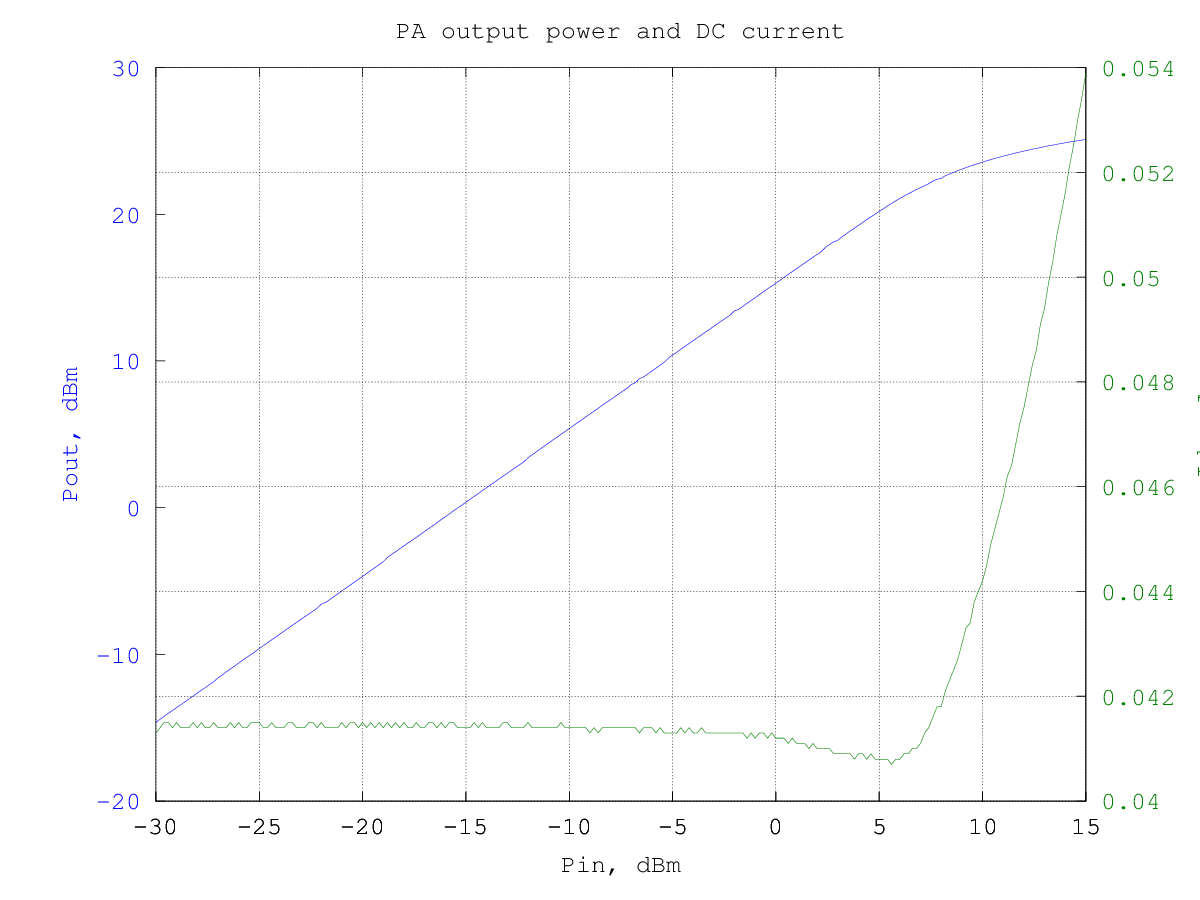and the resulting efficiency is shown in the graphs below (data are the same for the two graphs, in the first the input and output power are in dBm and in the second they are in watt):

The third-order intermodulation products amplitude w.r.t the output power per-tone is shown in the graph below (to obtain the actual [average] output power multiply the value by two, to have the PEP, multiply by 4):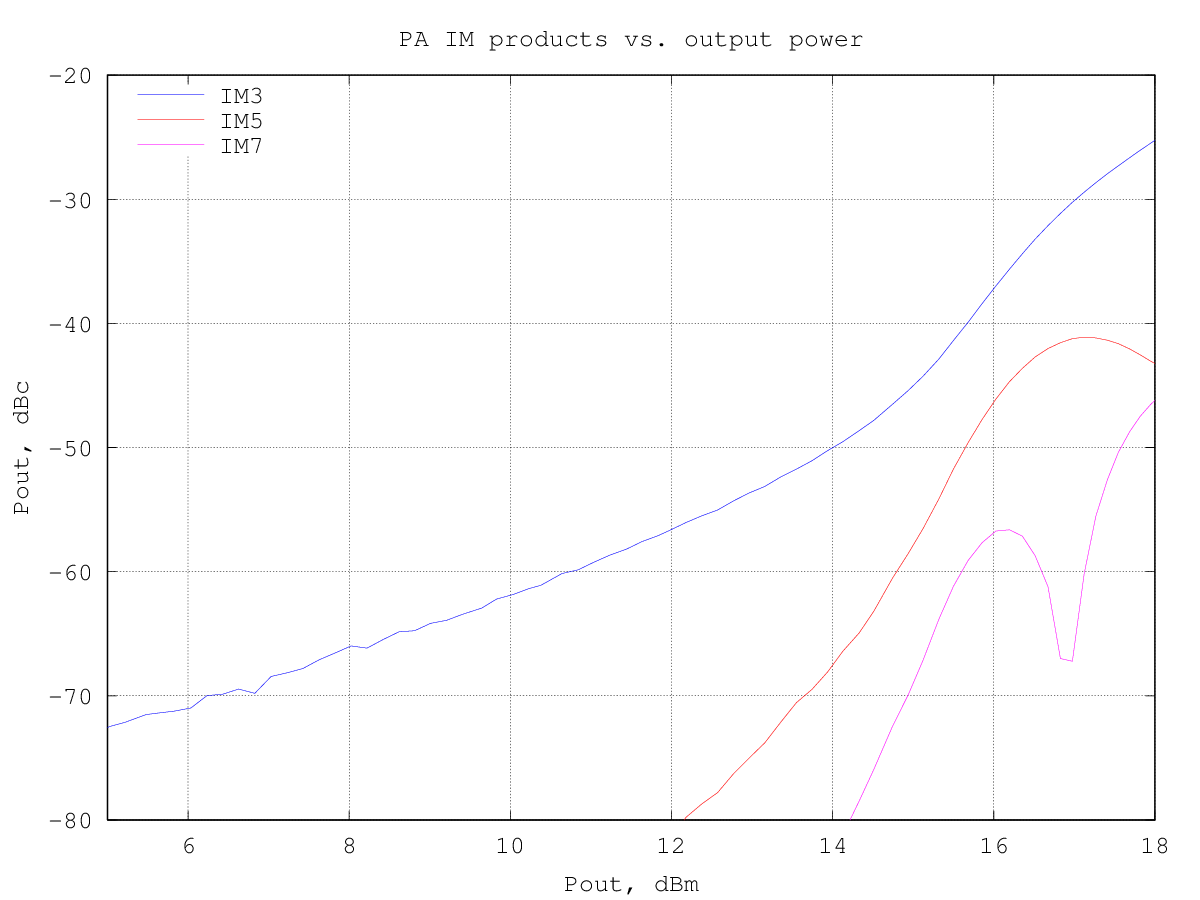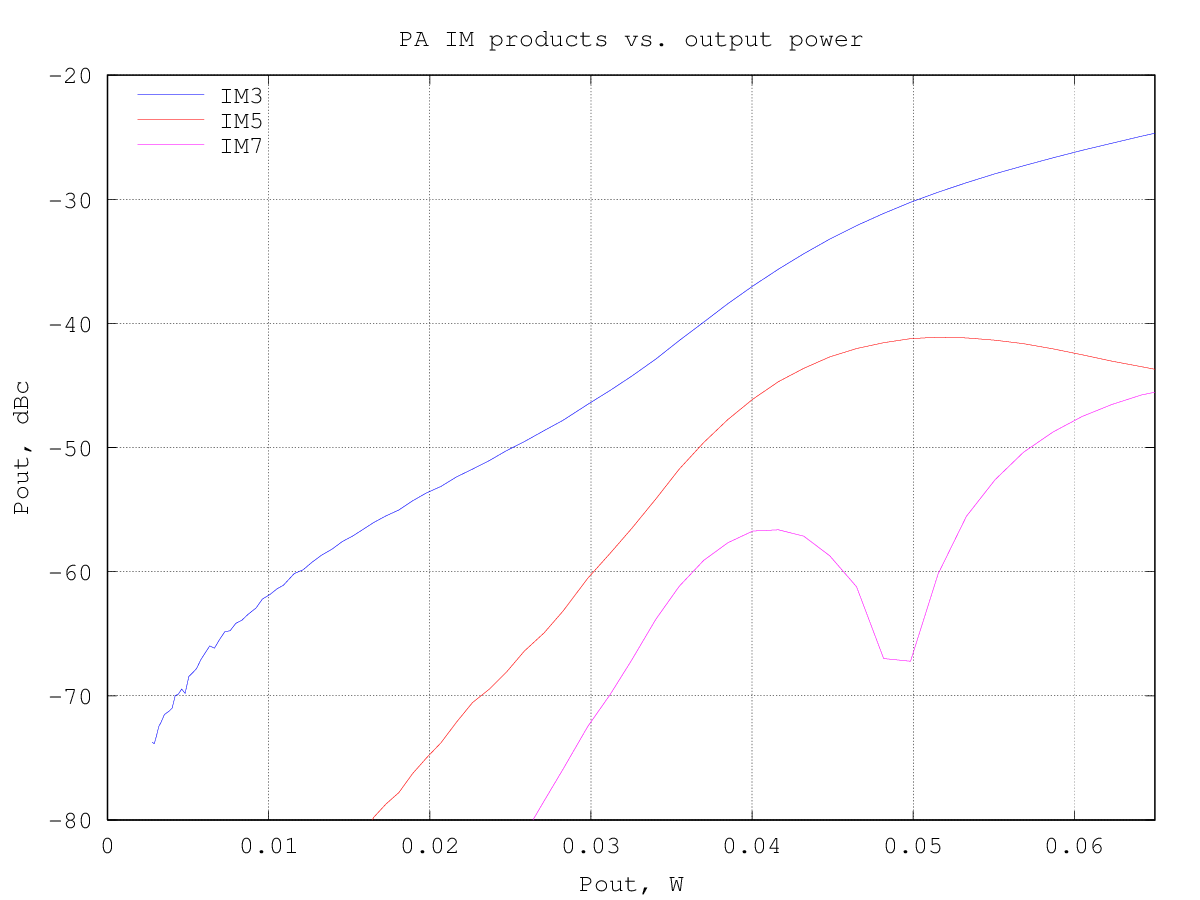at an IM3 of -30 dBc the output power is around 50 mW per tone.

The graph below shows the relative amplitude of the IMD products (separately for upper and lower products) for a two-tone test with varying tone spacing at 195 mW PEP output:By plotting the third-order intermodulation products power vs. the input power we can find the intercept point by extrapolating the results. The amplifier third-order intermodulation products follow the theoretical 3:1 slope w.r.t. the input power up to about -5 dBm input, as can be seen from the graph below: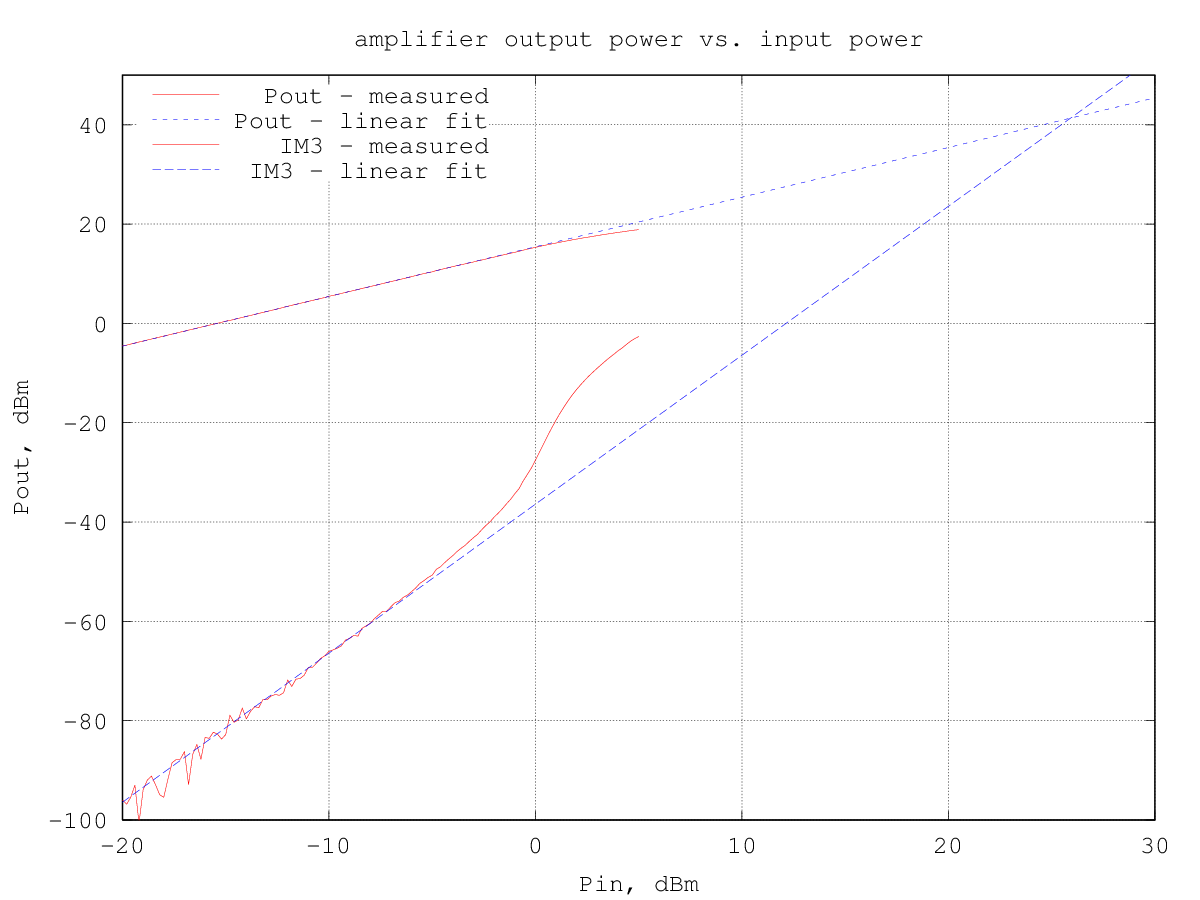The input IP3 turns out to be 25.9 dBm and the corresponding output IP3 is 41.3 dBm.

## Second version, with a 2SC5551A

All measurement made with Vdc=12.0 V. IccQ=38 mA.

The schematic is as for the first version above, except for the active device, which is now a 2SC5551A.
Quite surprisingly, the amplifier did not show any parasitic oscillation, which I was expecting, due to the high fT of this device.

The amplifier was built using the previous circuit, just changing the active device. Since the power dissipated is not low, an improvised heatsink was soldered to the transistor collector tab: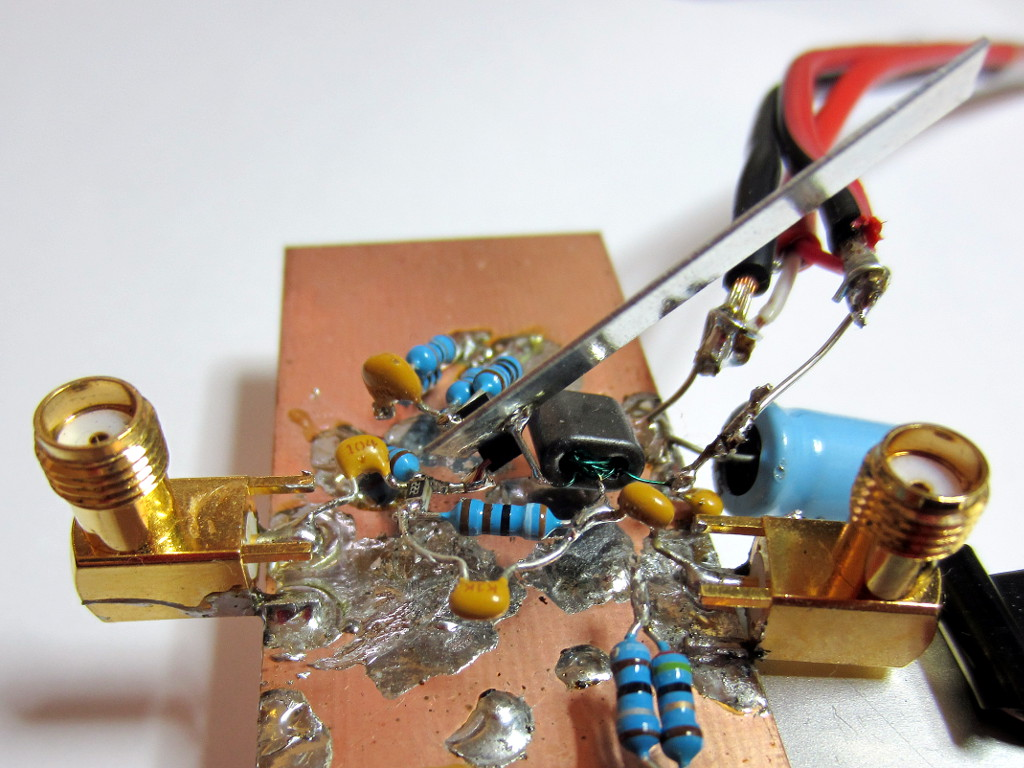Intermodulation products at 160 mW PEP output: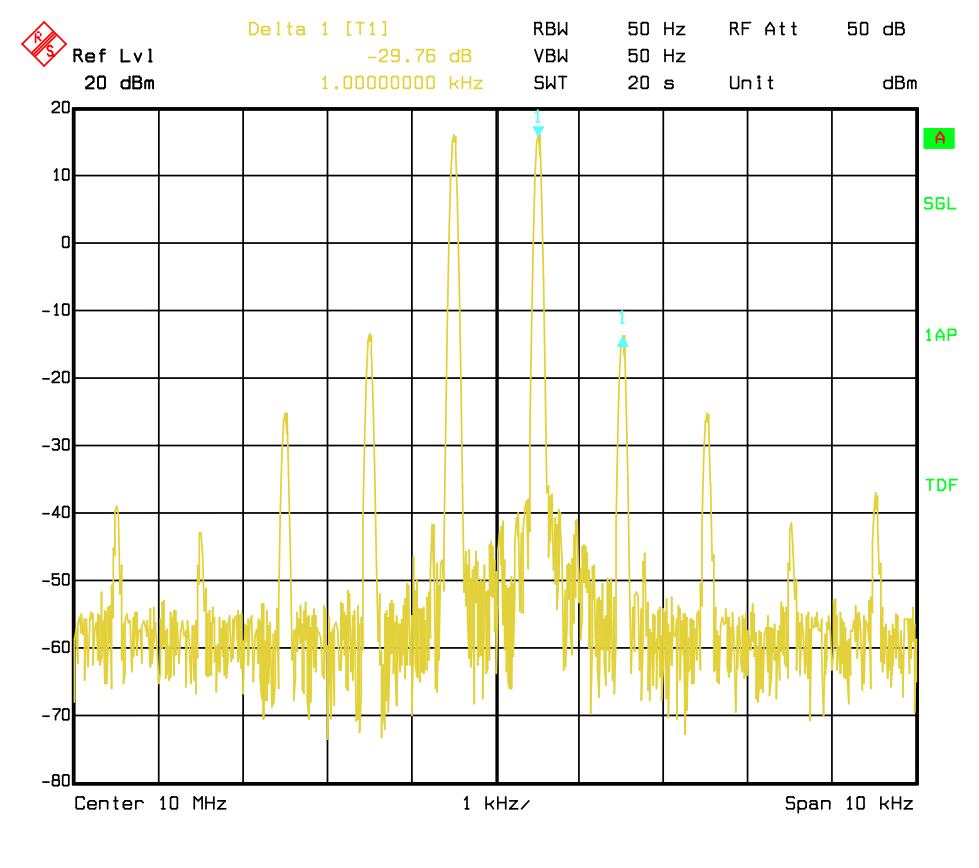Measured small-signal S-parameters (with -16 dBm input):.

The fundamental and harmonics output levels versus input power, with a 10 MHz sinewave input, is shown in the graph below: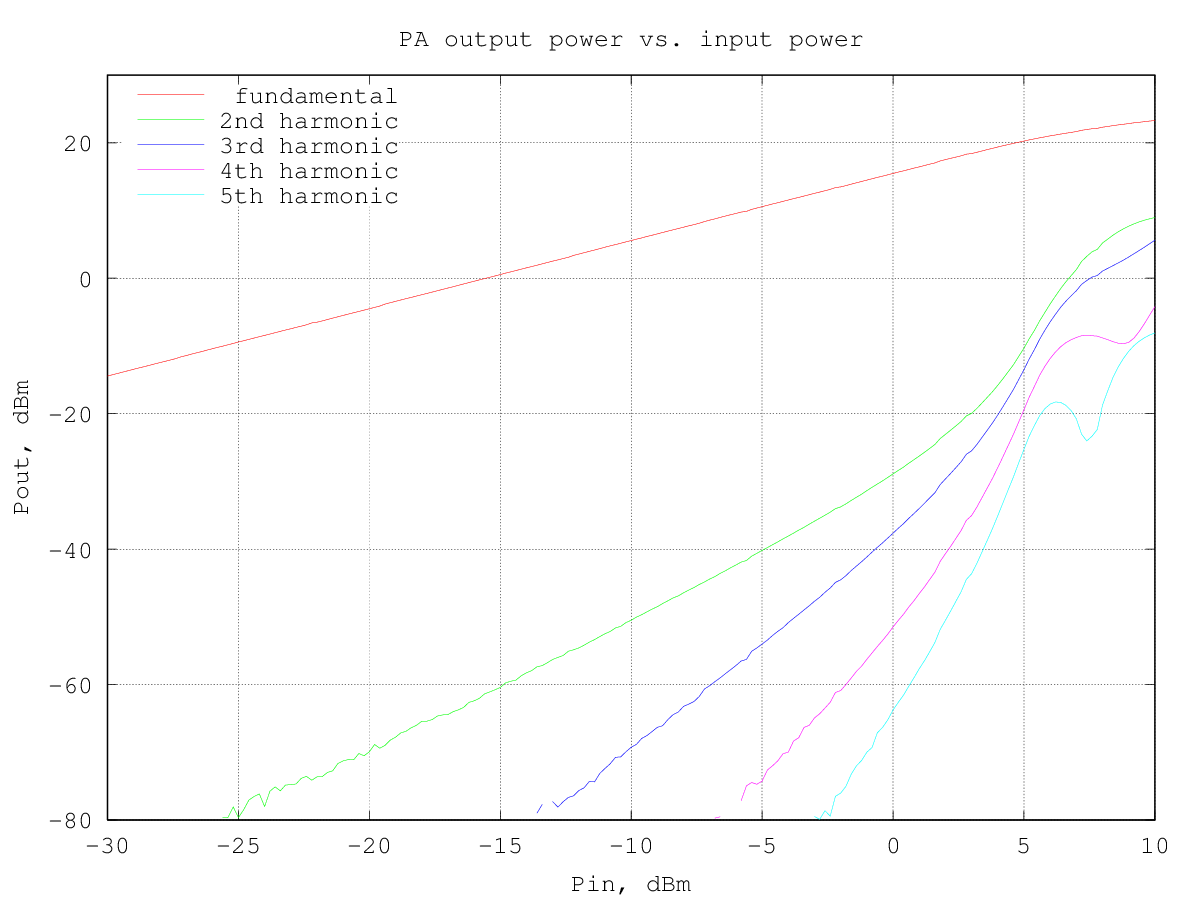the output power at 1 dB of compression is about 160 mW (22.0 dBm) - slightly less than the 2N2222A version, maybe because of the slightly lower bias current.

RF output and DC current drawn from the supply versus input power (10 MHz sinewave) are shown in the following graph: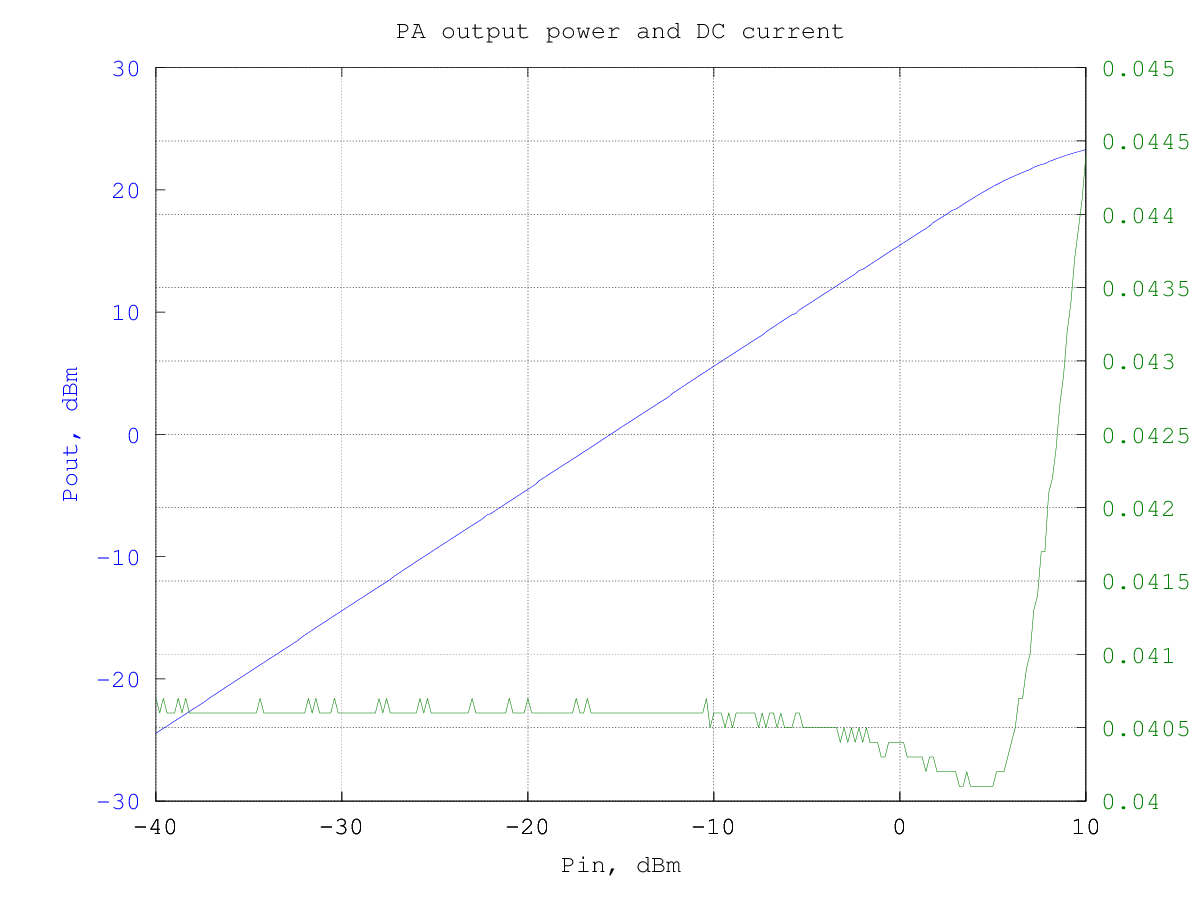and the resulting efficiency is shown in the graphs below (data are the same for the two graphs, in the first the input and output power are in dBm and in the second they are in watt):

The third-order intermodulation products amplitude w.r.t the output power per-tone is shown in the graph below (to obtain the actual [average] output power multiply the value by two, to have the PEP, multiply by 4):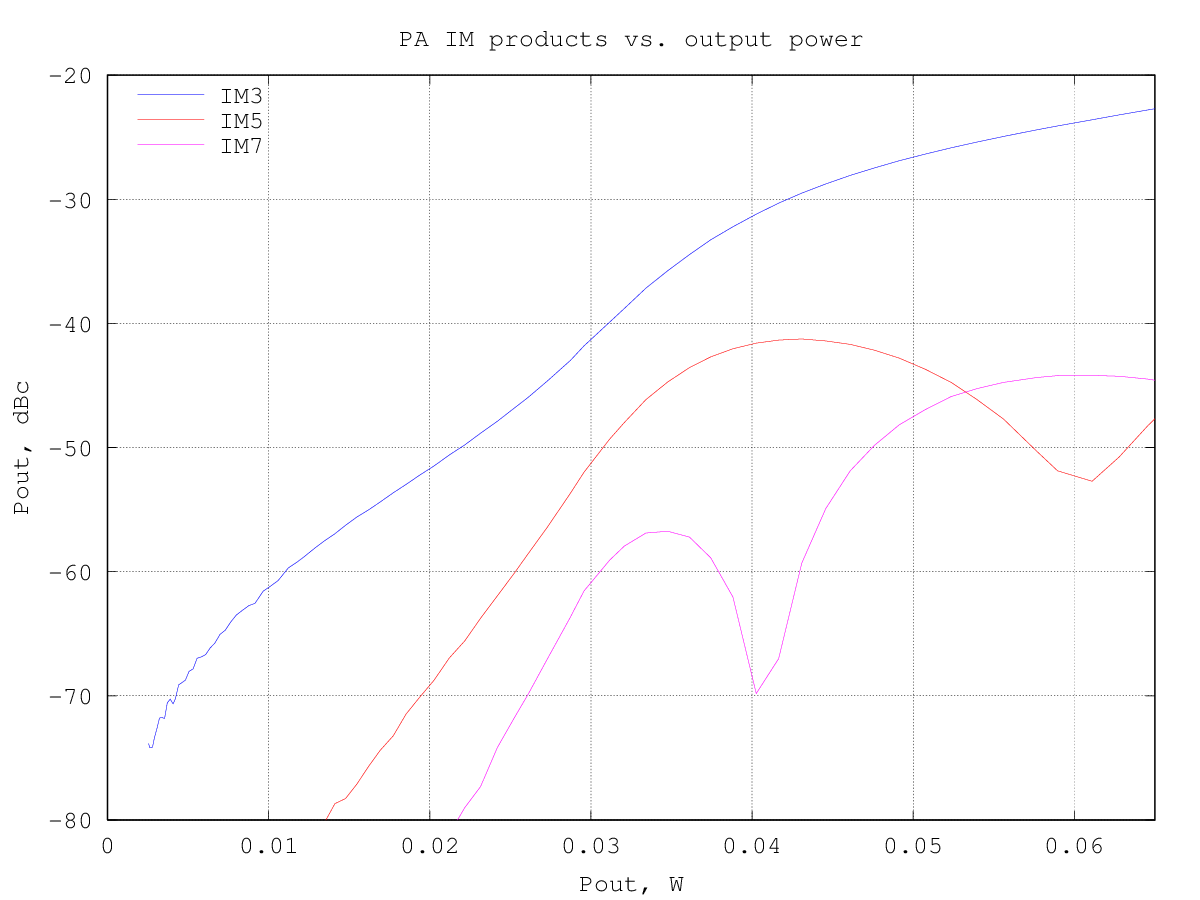at an IM3 of -30 dBc the output power is around 42 mW per tone.

The graph below shows the relative amplitude of the IMD products (separately for upper and lower products) for a two-tone test with varying tone spacing at 170 mW PEP output:By plotting the third-order intermodulation products power vs. the input power we can find the intercept point by extrapolating the results. The amplifier third-order intermodulation products follow the theoretical 3:1 slope w.r.t. the input power up to about -5 dBm input, as can be seen from the graph below:The input IP3 turns out to be 25.6 dBm and the corresponding output IP3 is 41.1 dBm, practically the same as the 2N2222A version.